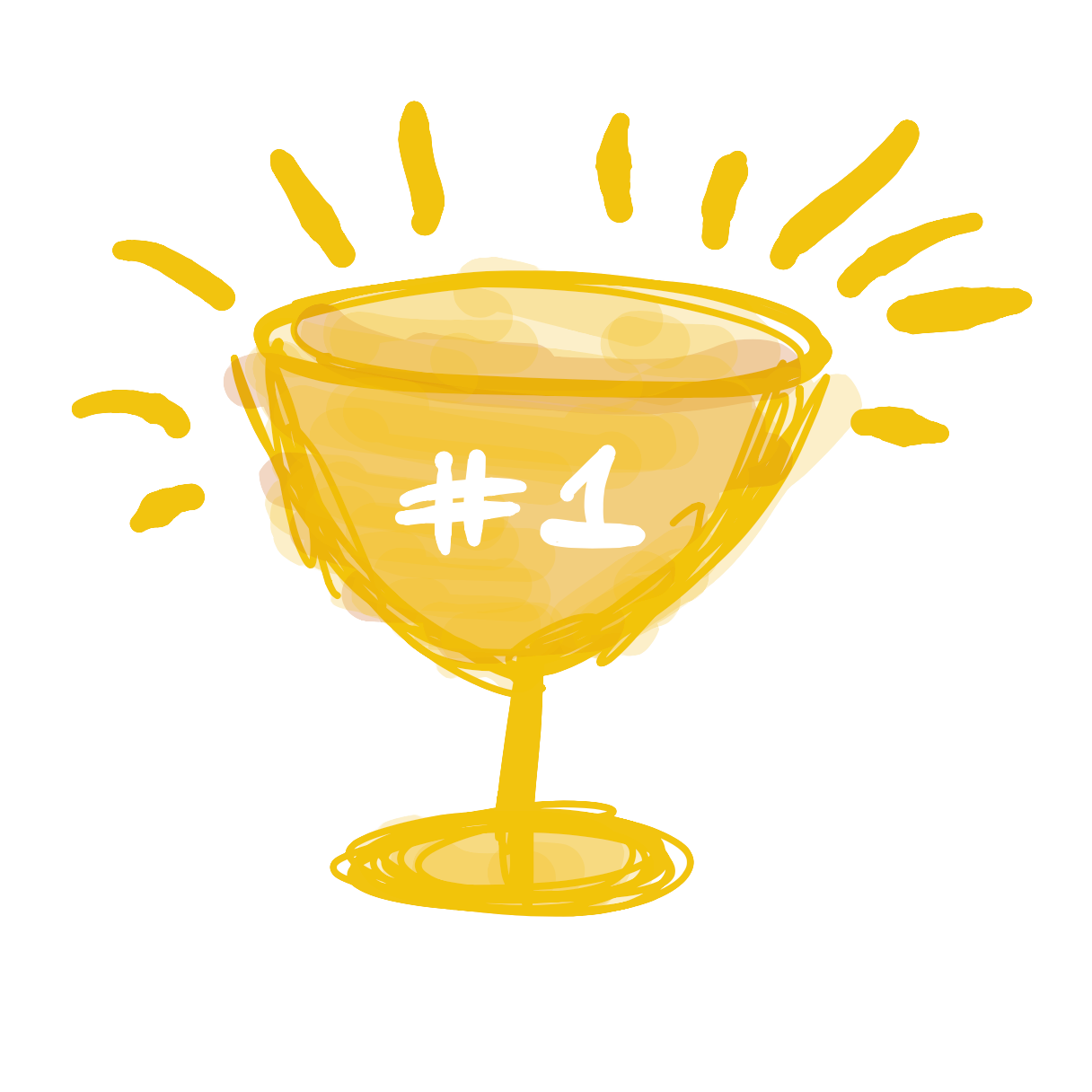# Animations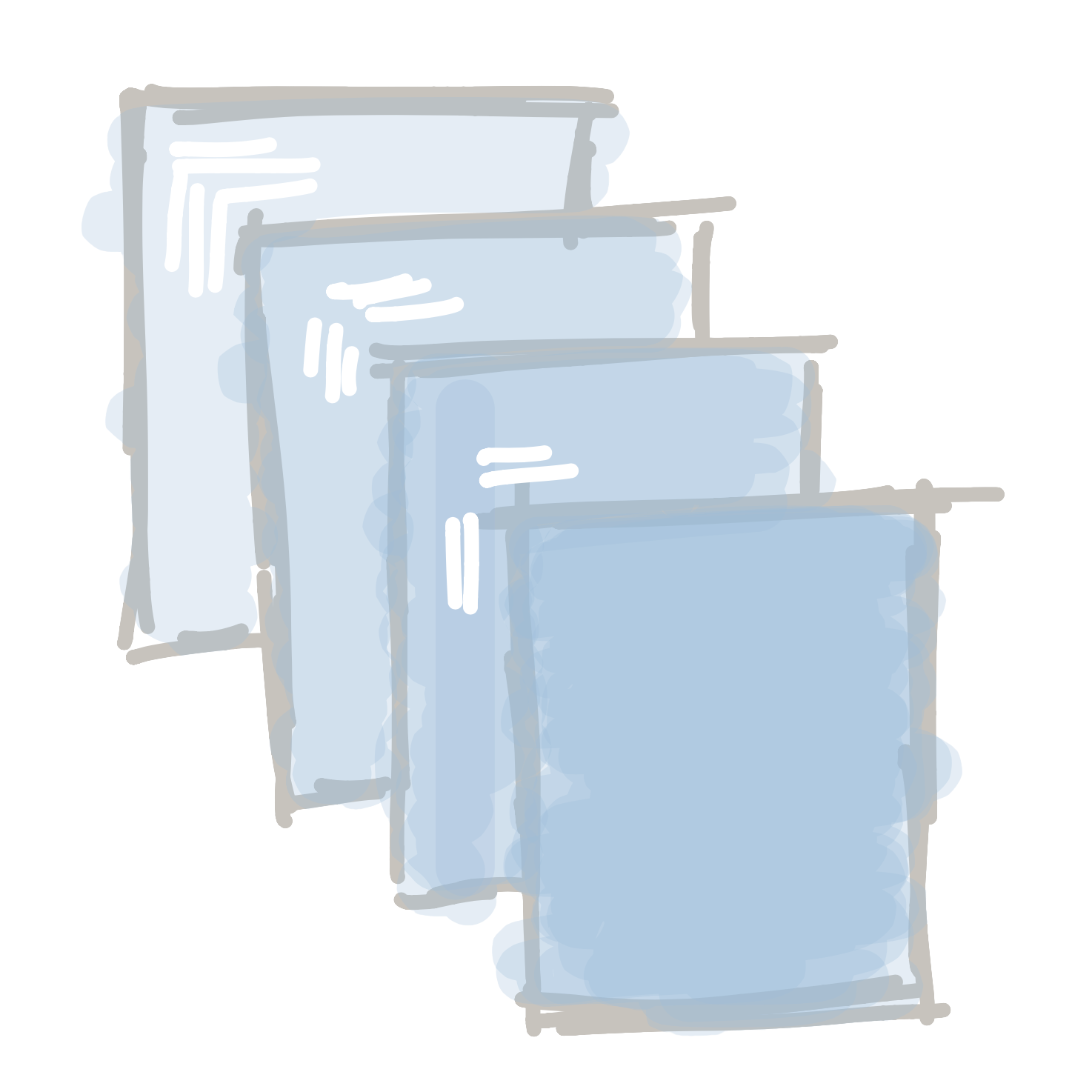Up till now, all of programs have been un-moving pictures. But the whole point of a program is being able to make something that can move and react to things. Let’s start bringing our programs to life!

Let’s start by looking into how animations work. The first every animation was this short film: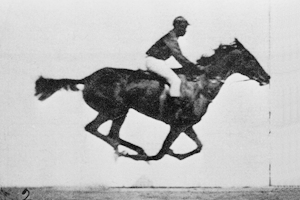The movie was actually a collection of 16 different pictures that were played one after the other, really quickly:This is still how we animate things on screen - either for movies or when we are making a program. We draw different pictures really quickly, and our eyes turn them into animations.

To animate things, we need to use two new functions. Let’s introduce ourselves to `void setup() {}` and `void draw() {}`: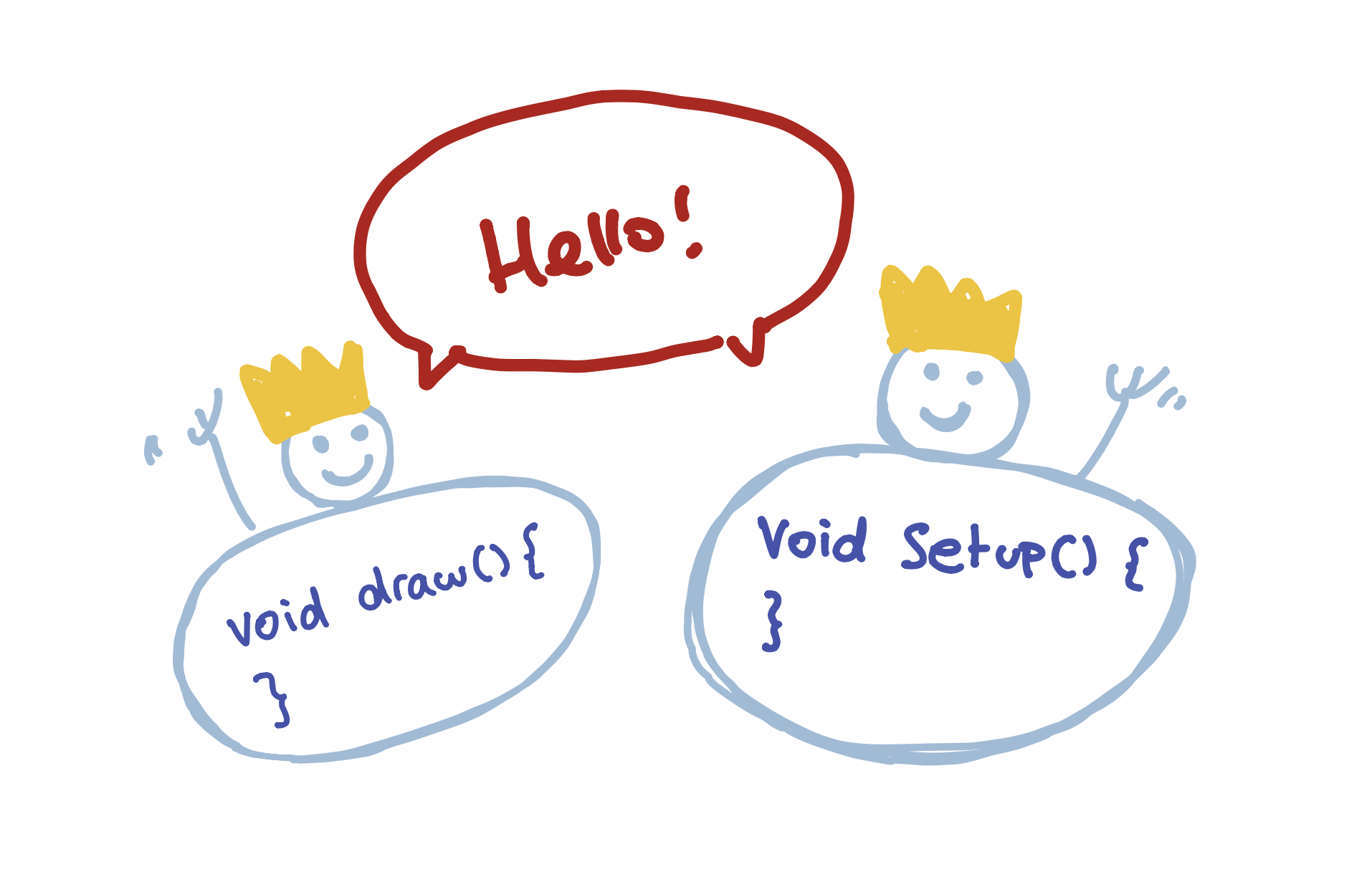These are functions just like the other ones we’ve used (ellipse(), rect(), our custom face() function, and more…), but they’re slightly special (that’s why they have crowns on their head). These functions are special because they are called automatically be the program. They also act a little differently.

The `void setup() {}` function is called once, at the very start of the program. If we need to do anything once, this is where that code will go: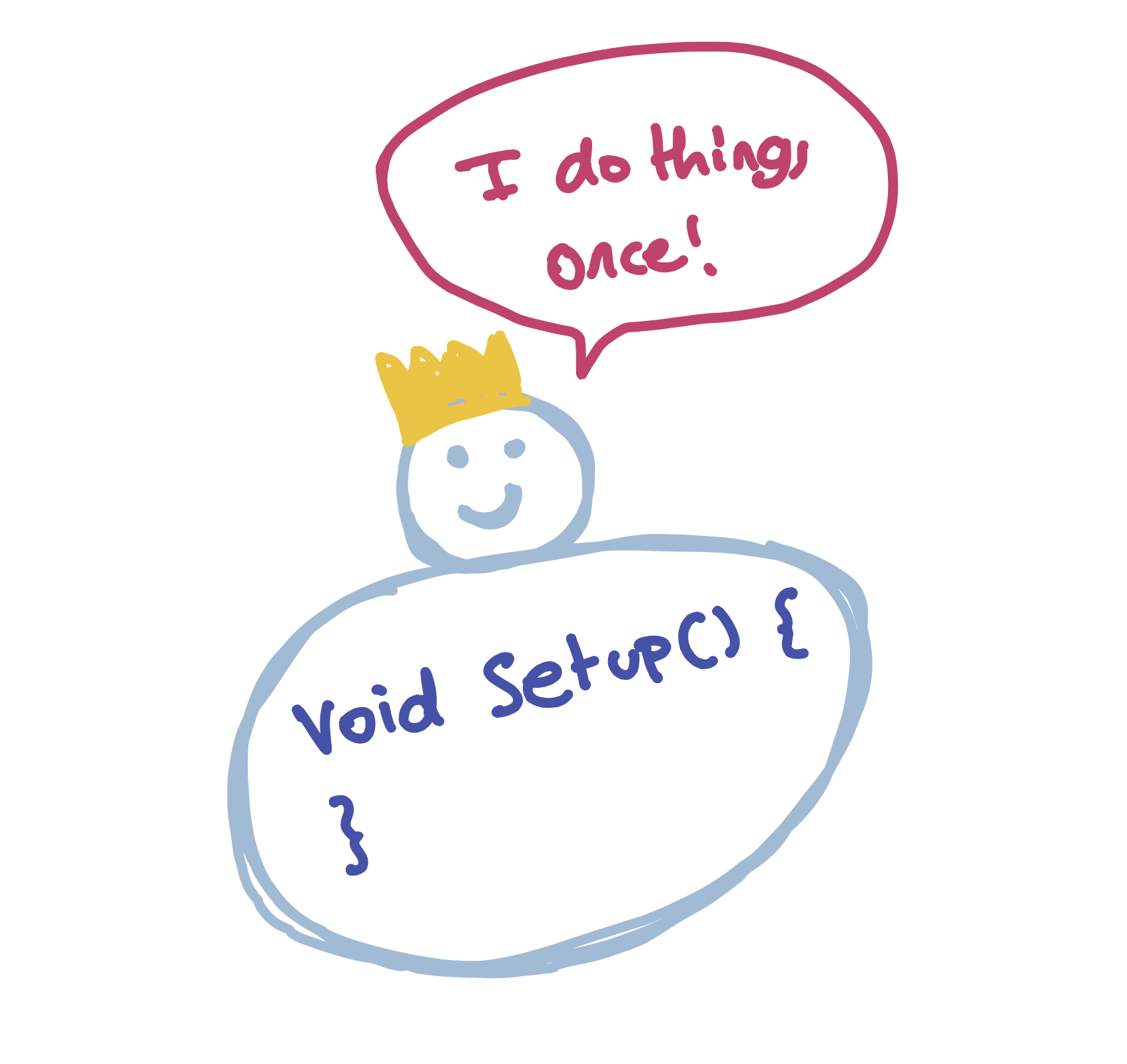The `void draw() {}` function is called 30 times a second. This is where all of our animation code will go: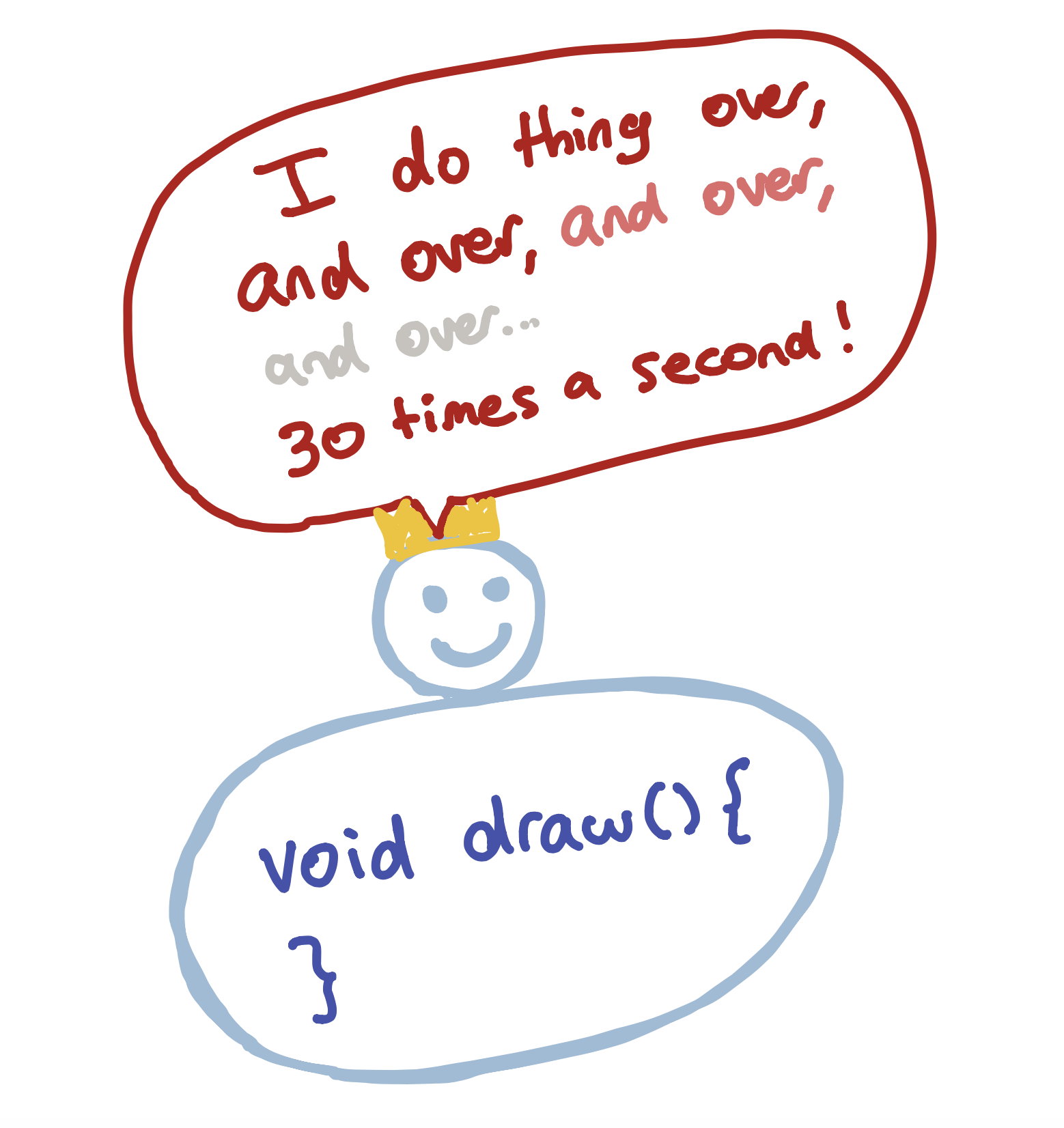Let’s take our face function…

##### Code:
```void setup() {
size(300,100);

int left = 20;
int eyeSize = 20;

face(left, eyeSize);
}

void face(int left, int eyeSize) {
ellipse(left + 5,30,eyeSize,eyeSize);
ellipse(left + 50,30,eyeSize,eyeSize);
triangle(left + 30, 40, left + 25, 50, left + 35, 50);
rect(left,60,60,20);
}

```
##### Preview:

… and convert it into our first animated program:

##### Code:
```void draw() {
int left = 20;
int eyeSize = 20;
face(left, eyeSize);
}

void setup() {
size(300,100);
}

void face(int left, int eyeSize) {
ellipse(left + 5,30,eyeSize,eyeSize);
ellipse(left + 50,30,eyeSize,eyeSize);
triangle(left + 30, 40, left + 25, 50, left + 35, 50);
rect(left,60,60,20);
}
```
##### Preview:

Pretty underwhelming, huh? A lot changed, but the program doesn’t look any different. So what did we just do?

The first thing we did is that we changed the `void setup() {}` function to `void draw() {}`. This means that the code inside gets run 30 times a second instead of once.

Next, we created a new `void setup() {}` function and moved the `size(300,100);` function into that. We have to move the size function into `setup()`, because Processing does not let us resize the screen; this function can only be called once at the start of the program.

The reason the program isn’t animating is because nothing is changing each time the `draw()` function is called. We are actually drawing a new face over and over, but it’s always in the same place and so it doesn’t look any different.

If we want to change what is shown on screen, we need to start changing some variables. But we need to think a little bit about how we do this. If we were to just add a `left += 1;` line to our `draw()` function, nothing would change:

##### Code:
```void draw() {
int left = 20;
int eyeSize = 20;
left += 1;
face(left, eyeSize);
}

void setup() {
size(300,100);
}

void face(int left, int eyeSize) {
ellipse(left + 5,30,eyeSize,eyeSize);
ellipse(left + 50,30,eyeSize,eyeSize);
triangle(left + 30, 40, left + 25, 50, left + 35, 50);
rect(left,60,60,20);
}
```
##### Preview:

This is because we re-create the `left` variable each time the `draw()` function is called. We first create it with a value of 20, then we add 1, then we draw the face, then we re-create it with a value of 20, add 1, draw the face, etc. etc.

So if we want to change the value of `left` each time `draw()` is called, we have to create the variable once - outside of the draw function:

##### Code:
```int left = 20;
int eyeSize = 20;

void draw() {
left += 1;
face(left, eyeSize);
}

void setup() {
size(300,100);
}

void face(int left, int eyeSize) {
ellipse(left + 5,30,eyeSize,eyeSize);
ellipse(left + 50,30,eyeSize,eyeSize);
triangle(left + 30, 40, left + 25, 50, left + 35, 50);
rect(left,60,60,20);
}
```
##### Preview:
Tip

We don’t put the left and eyeSize variables in the setup() function because variables that are created inside a function can only be seen in that function. If we want to create a variable that is used in different functions, we have to create it outside.

Our animation is cool, but why is there a trail to the left of our face? This is because every time `draw()` is called, it draws new things on top of whatever was on the screen before. If we want to clean the screen before we redraw the face, we need to tell the computer to do so. This is actually really easy to do, and you’ve already used the function to do it. All we need to do is tell the computer to set a new background color before redrawing anything, like this:

background(180);

So our final animation will look something like this:

##### Code:
```int left = 20;
int eyeSize = 20;

void draw() {
background(180);
left += 1;
face(left, eyeSize);
}

void setup() {
size(300,100);
}

void face(int left, int eyeSize) {
ellipse(left + 5,30,eyeSize,eyeSize);
ellipse(left + 50,30,eyeSize,eyeSize);
triangle(left + 30, 40, left + 25, 50, left + 35, 50);
rect(left,60,60,20);
}
```
##### Preview:
Next lesson Let's make a game